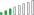cancel
Showing results for
Did you mean:Helper IV

I would like to use DAX to insert a header which shows what filter selections have been made.  It need to show "Level (Group): " followed by the filter selection.  If there's more than one selection then to show them all separated by ", " and if there are no filter selections then to just show the following text, "Level (Group): All".

My DAX is...

IF (
ISFILTERED ( 'Austrade (Append)'[Level (groups)] ),
"Level (Group): "
& CONCATENATE (
ALLSELECTED ( 'Austrade (Append)'[Level (groups)] ),
"Level (Group): All"
)
)

This is what it gives me though.Any tips would be gratefully received.

Best,

DHB

2 ACCEPTED SOLUTIONSSuper User

Give this a try

``````Commencing Header =
IF (
ISFILTERED ( 'Austrade (Append)'[Level (groups)] ),
VAR __f = FILTERS ( 'Austrade (Append)'[Level (groups)] )
VAR __d = CONCATENATEX ( __f, 'Austrade (Append)'[Level (groups)], ", " )
VAR __x = "Level (Group): " & __d
RETURN __x,
"Level (Group): All"
)``````Super User

Yes, we can add a count of the total number of items and compare the selection to that.

``````Commencing Header =
IF (
ISFILTERED ( 'Austrade (Append)'[Level (groups)] ),
VAR __f = FILTERS ( 'Austrade (Append)'[Level (groups)] )
VAR __t = COUNTROWS ( CALCULATETABLE ( DISTINCT ( 'Austrade (Append)'[Level (groups)] ), ALL ( 'Austrade (Append)' ) ) )
VAR __d = CONCATENATEX ( __f, 'Austrade (Append)'[Level (groups)], ", " )
VAR __x = IF ( COUNTROWS ( __f ) = __t, "Level (Group): All", "Level (Group): " & __d)
RETURN __x,
"Level (Group): All"
)``````
6 REPLIES 6Super User

Yes, we can add a count of the total number of items and compare the selection to that.

``````Commencing Header =
IF (
ISFILTERED ( 'Austrade (Append)'[Level (groups)] ),
VAR __f = FILTERS ( 'Austrade (Append)'[Level (groups)] )
VAR __t = COUNTROWS ( CALCULATETABLE ( DISTINCT ( 'Austrade (Append)'[Level (groups)] ), ALL ( 'Austrade (Append)' ) ) )
VAR __d = CONCATENATEX ( __f, 'Austrade (Append)'[Level (groups)], ", " )
VAR __x = IF ( COUNTROWS ( __f ) = __t, "Level (Group): All", "Level (Group): " & __d)
RETURN __x,
"Level (Group): All"
)``````Helper IV

Thank you @jdbuchanan71 that is totally awesome.Super User

Give this a try

``````Commencing Header =
IF (
ISFILTERED ( 'Austrade (Append)'[Level (groups)] ),
VAR __f = FILTERS ( 'Austrade (Append)'[Level (groups)] )
VAR __d = CONCATENATEX ( __f, 'Austrade (Append)'[Level (groups)], ", " )
VAR __x = "Level (Group): " & __d
RETURN __x,
"Level (Group): All"
)``````Helper IV

That's perfect, thank you @jdbuchanan71 .  If someone manually clicked all 5 to get all, rather than not selecting any, is there something I could add to that code to make it read "Level (Group): All" instead of getting a concatented list of all 5?Resolver III

Check if you only have one value and then return that one value, if more than one value use the following.

``CONCATENATEX('Austrade(Append)', 'Austrade(Append)'[Level (Groups)], ",") ``Solution Sage